# Rotate an Array by K Position using Reversal Algorithm in C#

## Rotate an Array by K Position using Reversal Algorithm in C#

In this article, I am going to discuss Rotate an Array by K Position using Reversal Algorithm in C# with Examples. Please read our previous article where we discussed Rotating an array by K position using Popup and Unshifting Algorithm in C#. Rotation of an array basically means shifting each and every element to specified positions to the left or right.

##### Rotation of Array using Reversal Algorithm in C#

The Reversal Algorithm is an algorithm to rotate an array by position k with time complexity 0(n) and in place (which means no other array needs to be created).

##### The Logic of the Reversal Algorithm
###### Step1:

Suppose the position of rotation is 3 and an input array is: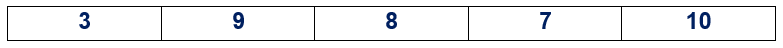In this algorithm, the array will be divided into two groups say group 1 and group 2.
Group 1 will be from 0 to (position -1)
Group 2 will be from the position to (n-1)
Where n denotes the length of the array and position denotes the position of the rotation.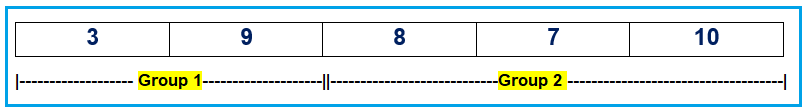###### Step2:

After dividing the whole array, we need to swap the values of each group with each other.
Group1: arr will be swapped with arr
Group2: arr will be swapped with arr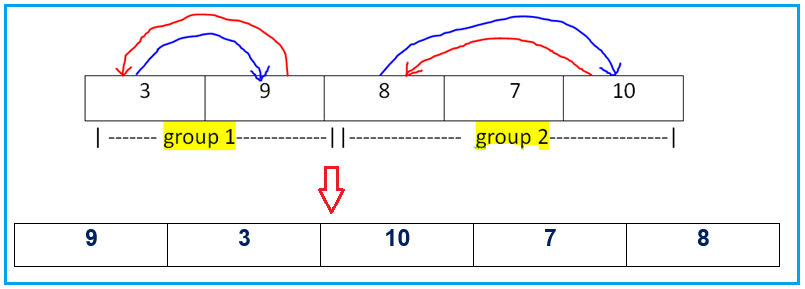###### Step3:

The whole array will be swapped once to get the rotation of the array as output.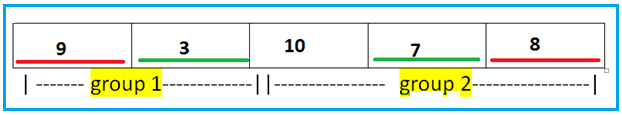Arr will be swapped with Arr
Arr will be swapped with Arr
After performing these 3 simple steps we will get the rotated array as output.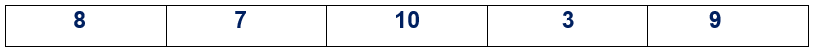###### Logic to rotate an array by using Reversal Algorithm
1. Take the value of position from the user.
2. Create a function named swapArray to swap the value with input parameters are array, starIndex, and lastIndex.
3. Call function swapArray with (arr, 0, (position-1))
4. Call function swapArray with (arr,position,(n-1))
5. Call function swapArray with (arr, 0, (n-1))
###### Example:

The following C# Program performs rotation of array by position k using reversal algorithm.

```using System;
namespace DotNetTutorials
{
public class ReversalAlgorithm
{
static void Main(string[] args)
{
int[] arr = { 1, 2, 3, 4, 5, 6, 7 };
Console.WriteLine("Original Array is :");
for (int i = 0; i < arr.Length; i++)
Console.Write(arr[i] + " ");

Console.WriteLine();

Console.Write("Enter value of k:");
ReversalAlgorithm obj = new ReversalAlgorithm();
obj.SwapElements(arr, 0, (position - 1));// 1st part
obj.SwapElements(arr, position, (arr.Length - 1));//2nd
obj.SwapElements(arr, 0, (arr.Length - 1));

Console.WriteLine();

Console.WriteLine("Rotation of array by position " + position);
for (int i = 0; i < arr.Length; i++)
Console.Write(arr[i] + " ");

}

//3 times
void SwapElements(int[] arr, int startIndex, int endIndex)
{
while (startIndex < endIndex)
{
int temp = arr[startIndex];
arr[startIndex] = arr[endIndex];
arr[endIndex] = temp;

startIndex++;
endIndex--;
}
}
}
}
```
###### Output: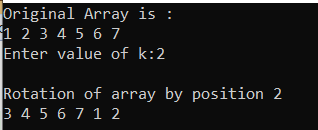In this article, I am going to discuss How to Reverse an Array in C# with Examples. Here, in this article, I try to explain Rotate an array by K position using Reversal Algorithm in C# with Examples and I hope you enjoy this Rotate an array by position k by using Reversal Algorithm in C# article.